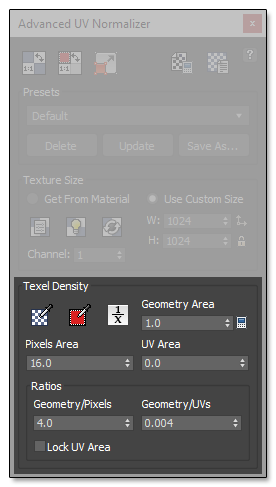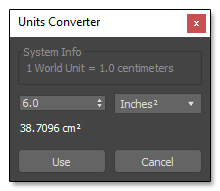## Texel DensityIn this section is where the Texel Density used in the Normalization process is defined.

You can get the average Texel Density from all the selected objects or just from the selected faces to be used later.

### Normalize Geometry/Pixels Areas

This will set the Geometry Area to 1.0 and change the Pixels Area to keep the same Ratio. It is useful if you get the Texel Density from an object and want to know the amount of pixels used per Geometry Unit.

### Geometry Area

It is the Area of the Geometry expressed in the Current Units System.

### Pixels Area

The current Pixel Area used by the Geometry Area. It is based on the specified Texture Size.

### UV Area

It is the UV Area used by all the UV Shells, expressed in UV Units.

### Geometry/Pixels Ratio

It is the Ratio between the Geometry and the used Texture Pixels. It represents the squared root of the Pixels Area per Geometry Unit. For example, a ratio of 4.0 means that 16 (4x4) pixels will be used per Geometry Unit, for a ratio of 8.0 a 64 (8x8) pixels area will be used per Geometry Unit, and so on.

Very small values might be trimmed on the spinners, depending on your 3ds Max setup. If you place the cursor over the spinner's arrows, the real value will be displayed in the tooltip.

### Units ConverterIt is a small utility to help you convert Geometry units to the current Units System used by your scene.